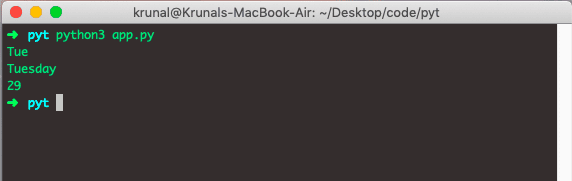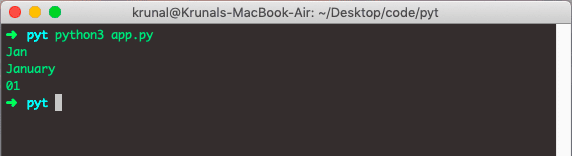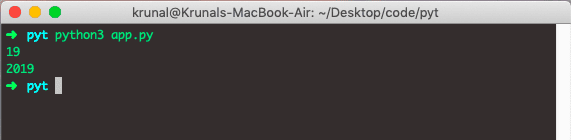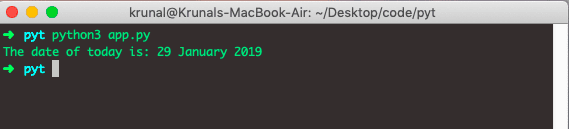# Python Date Format: The Complete Guide

Python has a datetime module with different class methods to change the date format. The date and datetime are the objects in Python. So when you manipulate them, you use objects, not string or timestamps. The date, time, and datetime classes provide some function to deal with the date, times, and time intervals.

Whenever you manage dates or times, you need to import the datetime function. If you are new to Python’s Date and Time chapter, check out my Datetime article on this blog.

## Python Date Format

The datetime classes in Python are categorized into five main classes.

1. date – Manipulate just date ( Month, day, year).
2. time – Time independent of the day (Hour, minute, second, microsecond).
3. datetime – Combination of time and date (Month, day, year, hour, second, microsecond).
4. timedelta— A duration of time used for manipulating dates.
5. tzinfo— An abstract class for dealing with time zones.

## Formatting Date: Days

Let’s display the three variations of the Day. For this example, I am using a Current Date.

Create a file called app.py and add the following code.

```# app.py

from datetime import datetime

now = datetime.now()

print(now.strftime('%a'))
print(now.strftime('%A'))
print(now.strftime('%d'))```

In the above code, we have used the three abbreviations.

The first print statement prints the Tue, the second prints Tuesday, and the third prints 29.

See the following output.The % is a abbreviated day of the week: Mon, Tue, and Wed.

The %A is the full name of the day of the week: Monday, Tuesday, and Wednesday.

%d is the day of the month. This means today is the 29th of January. So it will print 29.

We have used the now object’s strftime function. So let’s see more detail on that function.

## Python strftime()

The strftime() method returns the string representing date and time using the date, time, or datetime object.

The strftime() method takes one or more formats as an argument and returns the formatted string based on it.

## Formatting Date in Python: Months

Let’s see the different variations of the months.

The %b  is the abbreviated month name like Jan, Feb, Mar

The %B stands for the full month name like January, February

The %m stands for a month as a number: 01  for January, 02 for February.

Let’s see this in action. First, write the following code inside the app.py file.

```# app.py

from datetime import datetime

now = datetime.now()

print(now.strftime('%b'))
print(now.strftime('%B'))
print(now.strftime('%m'))```

See the following output.## Formatting Date in Python: Years

Let’s see the different variations of the years.

The %y abbreviated years as two numbers: 19.

The %Y abbreviated years as four digits: 2019.

Let’s try the following code.

```# app.py

from datetime import datetime

now = datetime.now()

print(now.strftime('%y'))
print(now.strftime('%Y'))```

See the output.## Combine Date Format Variations

Write the code to combine different date format variations.

```# app.py

from datetime import datetime

now = datetime.now()
print('The date of today is:',now.strftime('%d %B %Y'))```

Let’s see the following output.So, we have seen almost all the variations in Day, Month, and Year.

That’s it for this tutorial.

This site uses Akismet to reduce spam. Learn how your comment data is processed.